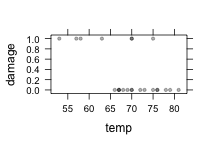# Monday 22 April 2013

### Working with Count Data and Odds Ratios

Create an example data set:

foo = data.frame(exposed = c("Y", "N", "Y", "N"), sick = c("Sick", "Sick", "Healthy",
"Healthy"))
foo

##   exposed    sick
## 1       Y    Sick
## 2       N    Sick
## 3       Y Healthy
## 4       N Healthy

goo = foo[c(rep(1, 10), rep(2, 20), rep(3, 2000), rep(4, 1000)), ]
tally(~sick | exposed, data = goo)

##          exposed
## sick             N        Y
##   Healthy 0.980392 0.995025
##   Sick    0.019608 0.004975
##   Total   1.000000 1.000000

glm(sick == "Sick" ~ exposed, data = goo, family = "binomial")

##
## Call:  glm(formula = sick == "Sick" ~ exposed, family = "binomial",
##     data = goo)
##
## Coefficients:
## (Intercept)     exposedY
##       -3.91        -1.39
##
## Degrees of Freedom: 3029 Total (i.e. Null);  3028 Residual
## Null Deviance:       337
## Residual Deviance: 323   AIC: 327


### Logistic Regression

When we do linear regression on a Zero/One variable, we are effectively modeling the probability.

• Easy to see if there is a single, categorical explanatory variable — the model value is just the groupwise means in the different levels of the variable.
• A bit more subtle if the explanatory variable is quantitative. Draw the picture with Zero/One response variable on the vertical axis and a quantitative variable on the horizontal axis. Example: O-ring damage in the space shuttle.
orings = fetchData("oring-damage.csv")

## Retrieving from http://www.mosaic-web.org/go/datasets/oring-damage.csv

xyplot(damage ~ temp, data = orings, pch = 20, col = rgb(0, 0, 0, 0.3))Sketch in the best fitting line. But a line is too “stiff” and needs eventually to escape 0-1.

Remember that the idea of logistic regression is to fit a linear model to the “log odds”, which is just the logarithm of $$p/(1-p)$$ — a different format for the probability. We haven't talked about how this is done. That's more advanced.

### Odds and the Odds Ratio

Suppose we have some data on an illness and exposure to a potential toxin

Sick Healthy
Exposed A B
Not Exposed C D

Among the exposed, the risk of being sick is $$A/(A+B)$$.

Among the unexposed, the risk of being sick is $$C/(C+D)$$.

The risk ratio is the … ratio of the two risks! It tells how much more likely you are to get the sickness if you are exposed.

Now suppose that the sickness is fairly rare, say roughly 1 in 1000. It's a huge amount of work to measure the exposure on everybody. Is it necessary, given that almost everybody is healthy.

To avoid this problem, it's common to do a case/control study, where we pick sick people from a clinic and a similar number of healthy controls. (Call up the friends of the sick kids. They will be similar in age, activities, etc.)

But now the ratio of A to B is wrong, it's roughly 1 to 1, whereas in the population it's roughly 1 to 1000

Instead, we calculate the odds and the odds ratio. $$\frac{A/C}{B/D}$$
Notice that if A is much less than B, and C is much less than D, the odds ratio is essentially the same as the risk ratio.

### The odds ratio in use

A study of bicycle helmet use and the influence of state law.

Demonstration of logistic regression and odds ratios. “Effects of state helmet laws on bicycle helmet use by children and adolescents” Injury Prevention 2002, 9:42-46

The coefficients in logistic regression correspond to log-odds ratios.

When they say “adjusted Odds Ratio”, they mean the odds ratio of one of the categorical variable levels relative to the reference level, with the other variables in the model as covariates. Notice that they give a confidence interval on the odds ratio itself. The calculation of this is straightforward given the standard error on the logistic regression coefficient.

Calculate the confidence interval on the odds ratio, e.g.

exp(0.7 + c(-1, 1) * 1.96 * 0.294)

##  1.132 3.583


## Power and Logistic Regression:

Aspirin and stroke.

The aspirin simulation gives a (not very detailed) model of the relationship between aspirin consumption and stroke. It has a confounder, sick, which represents how sick the patient is.

You can see the overall structure of the simulation:

fetchData("simulate.r")

## Retrieving from http://www.mosaic-web.org/go/datasets/simulate.r

##  TRUE

aspirin

## Causal Network with  3  vars:  sick, mgPerDay, stroke
## ===============================================
## sick is exogenous
## mgPerDay <== sick
## stroke <== sick & mgPerDay


The simulation itself is a little bit complicated. For what it's worth, you can look inside:

equations(aspirin)

## sick <== sample(c(rep(0,20),1:10,1:3,8:10), nsamps,replace=TRUE)
## mgPerDay <== 5*(sick<2)*sample(c(0,0,0,0:10,0:20),nsamps, replace=TRUE) + (sick>=2)*5*sample(c(0,0,0,0:20,10:20,15:20),nsamps,replace=TRUE)
## stroke <== cut(rnorm(nsamps,mean=0,sd=5) + 2*sick - mgPerDay/10, c(-Inf,5,Inf), labels=c('N','Y'))


Imagine that your research team has constructed this model. You've been asked to design the observational study. Two questions:

• Do you need to measure sick to get a meaningful result?
• How big should the study be to detect the beneficial influence of aspirin on stroke?

Assuming that it's important to include sick as a covariate, here's one trial in a power calculation for a study of size $$n=50$$.

f = run.sim(aspirin, 50)
summary(glm(stroke == "Y" ~ mgPerDay + sick, data = f))\$coef[2, 4]

##  0.08187


Generate many trials and find the power.

Then set the sample size as required to create a power of, say, 80%.

How much larger would the sample size need to be to create a power of 95%.

### Deer Crossing Project

See instructions at http://rpubs.com/dtkaplan/deer-crossing.